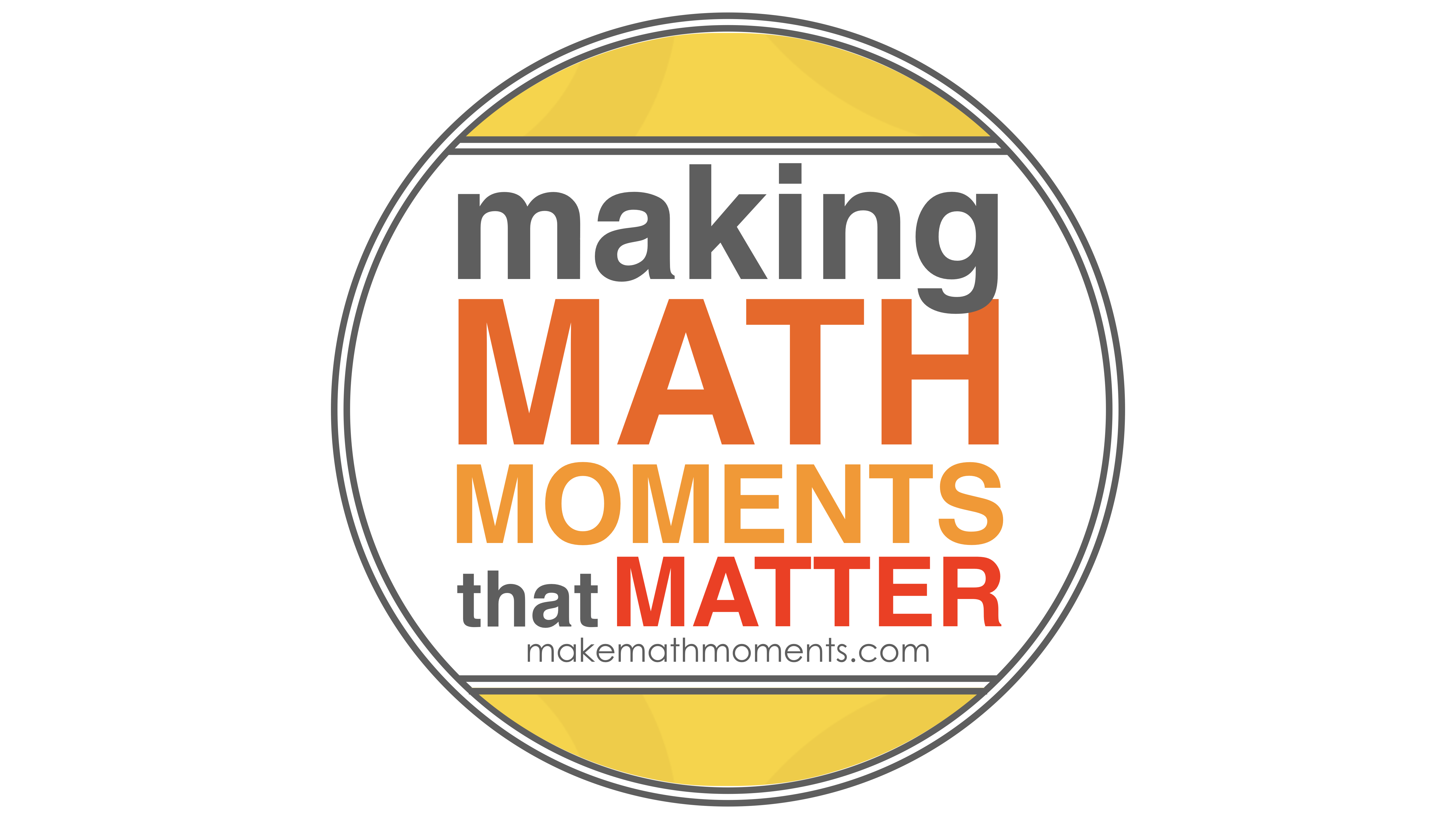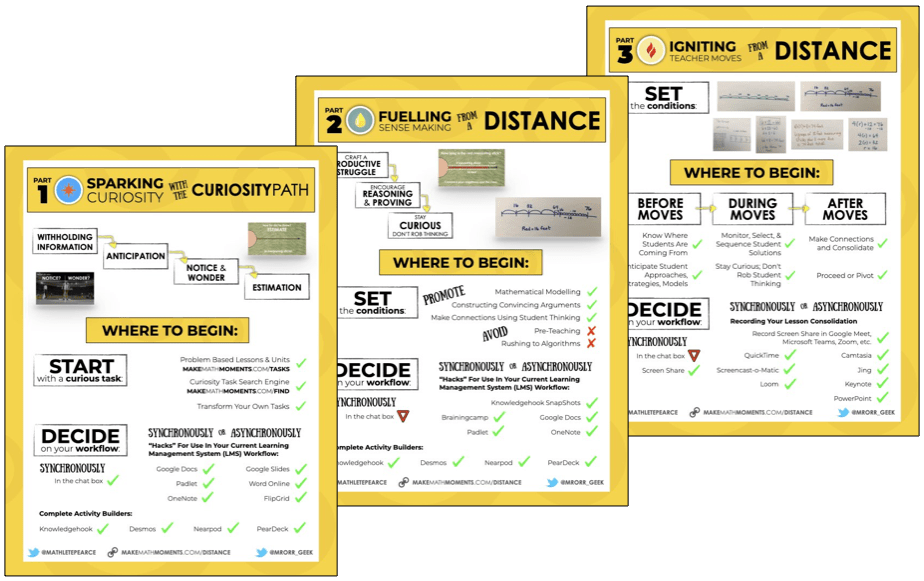# Playlist - Linear Models

## Using Number Paths As A Linear Model

In this series of Math Is Visual Prompts, we slowly introduce the idea of a linear model (i.e.: number line) through the use of a number path. This is a much less abstract way to introduce the idea of linear measurement through counting to make it accessible by all.

## Comparing and Ordering On A Number Path

In this series of Math Is Visual Prompts, we slowly introduce the idea of a linear model (i.e.: number line) by placing numbers on the number path.

## Solving One-Step Equations Visually

In this series of Math Is Visual Prompts, we slowly introduce the idea of Solving One-Step Equations Using Partitive and Quotative Division.

## Solving Two-Step Equations Visually

In this series of Math Is Visual Prompts, we slowly introduce the idea of Solving Two-Step Equations Using Partitive Division Scenarios.

## Solving Two-Step Equations Visually Part 2

In this series of Math Is Visual Prompts, we slowly introduce the idea of Solving Two-Step Equations Using Partitive and Quotative Division Scenarios.

## Magnitude Of Number: Where Does 1 Billion Go?

Explore magnitude of number by trying to determine where 1 billion should go on a number line from 0 to 1 trillion based on a Mark Chubb Tweet.

## Subtraction Using The Concrete Number Line

Explore comparison subtraction and separating subtraction (or take away subtraction) on the concrete number line using connecting cubes. Math is visual!

## Unitizing and Repeated Subtraction

Explore unitizing and repeated subtraction through this standardized test question made accessible through visuals. Math Is Visual: Let's Teach It That Way

GET MATH IS VISUAL PROMPTS TO YOUR INBOX!